# Fractions

Here you will learn about fractions, including different types of fractions, how to compare fractions, and how to operate with fractions.

Students will first learn about fractions as part of numbers and operations – fractions in 4th and 5th grade. They will continue to expand upon this knowledge in the number system in 6th grade.

## What are fractions?

Fractions are ways to show equal parts of a whole.

The denominator of a fraction (bottom number) shows how many equal parts the whole has been divided into.

The numerator of a fraction (top number) shows how many of the equal parts there are.

A proper fraction is a fraction where the numerator is smaller than the denominator.

For example,

2 equal parts 4 equal parts 12 equal parts

Step-by-step guide: Numerator and Denominator

Equivalent fractions are fractions that have the same value. You can use models or multiplication to find equivalent fractions.

For example,

What are two fractions equivalent to \, \cfrac{1}{3} \, ?

Step-by-step guide: Equivalent fractions

Two types of equivalent fractions that show wholes and parts are improper fractions and mixed numbers.

An improper fraction is a fraction where the numerator (top number) is larger than the denominator (bottom number).

For example,

A mixed number has a whole number and a fractional part

For example,

Any number greater than 1 can be shown as an improper fraction AND a mixed number.

For example,

\cfrac{3}{2} \, is 3 halves, which is one group of \, \cfrac{2}{2} \, and a group of \, \cfrac{1}{2} \, .

1\cfrac{1}{2} \, is 1 and one half, which is 1 whole and a group of \, \cfrac{1}{2} \, .

Step-by-step guide: Improper fraction to mixed number

Step-by-step guide: Mixed number to improper fraction

Comparing fractions is deciding whether one fraction is smaller than, larger than, or equal to another.

To do this, you can use common denominators, common numerators, or compare to benchmark fractions. Use inequality symbols < (less than) and > (greater than) to write the comparison.

For example,

Compare \, \cfrac{3}{4} \, and \, \cfrac{3}{8} \, .

• To use common denominators, multiply each fraction by the opposite denominator.

• To use common numerators, compare the denominators.

Fourths are larger, so 3 fourths will be larger than 3 eighths.

• To use benchmark fractions, compare each fraction to 0, \, \cfrac{1}{2} \, , and 1.

\cfrac{3}{8} \, is less than \, \cfrac{1}{2} \, , and \, \cfrac{3}{4} \, is right inbetween \, \cfrac{1}{2} \, and 1, so \, \cfrac{3}{4} \, is larger.

Step-by-step guide: Comparing Fractions

Ordering fractions is taking a set of fractions and listing them in ascending order (least to greatest) or descending order (greatest to least).

To do this, you use equivalent fractions to create common denominators and then compare the numerators.

For example,

Order \, \cfrac{2}{3}, \, \cfrac{3}{5} \, , and \, \cfrac{5}{6} \, from least to greatest.

All the denominators (3, 5 , and 6) have a multiple of 30, so you can use 30 as the common denominator.

\cfrac{2 \, \times \, 10}{3 \, \times \, 10}=\cfrac{20}{30} \quad \quad \cfrac{3 \, \times \, 6}{5 \, \times \, 6}=\cfrac{18}{30} \quad \quad \cfrac{5 \, \times \, 5}{6 \, \times \, 5}=\cfrac{25}{30}

From least to greatest, the fractions are:

\cfrac{18}{30}, \, \cfrac{20}{30}, \, \cfrac{25}{30} \,\, or \,\, \cfrac{3}{5}, \, \cfrac{2}{3}, \, \cfrac{5}{6} \, .

Step-by-step guide: Ordering fractions

Adding and subtracting fractions is when you operate with two or more fractions to find the difference or the total.

To do this, fractions need a common denominator (bottom number) to add or subtract. Then you add or subtract the numerators (top numbers) and keep the denominator the same.

For example,

\cfrac{7}{8}-\cfrac{3}{8}=

The equation is taking \, \cfrac{3}{8} \, away from \, \cfrac{7}{8} \, .

Since the denominators are the same, the parts are the same size. You subtract to see how many parts are left:

7-3 = 4

There are 4 parts. But what size are the parts? They are still eighths, so the denominator stays the same.

\cfrac{7}{8}-\cfrac{3}{8}=\cfrac{4}{8}

You can solve the addition version of this equation, \cfrac{7}{8}+\cfrac{3}{8}=\cfrac{10}{8} \, or 1 \cfrac{2}{8} \,, by adding the numerators instead of subtracting.

If the fractions have unlike denominators, you use equivalent fractions to create fractions with common denominators, then follow the same steps.

Step-by-step guide: Subtracting fractions

Step-by-step guide: Adding and subtracting fractions

Fractions of numbers are when we multiply a fraction by a whole number. The word “of” means to multiply.

For example,

\cfrac{1}{4} \times 12 is the same \, \cfrac{1}{4} \, of 12.

To solve with the equation, make the whole number an improper fraction: \, \cfrac{12}{1} \, .

Then multiply the numerators and denominators together:

\cfrac{1}{4} \times \cfrac{12}{1}=\cfrac{1 \, \times \, 12}{4 \, \times \, 1}=\cfrac{12}{4}=3

Step-by-step guide: Fraction of a number

Multiplying and dividing fractions is solving a multiplication or division equation where one or more of the numbers is a fraction.

For example,

\cfrac{1}{3} \times \cfrac{2}{3}

In the model, \, \cfrac{2}{3} \, is yellow, and \, \cfrac{1}{3} \, is blue. The product is where the fractions overlap in green.

The model shows \, \cfrac{2}{3} \, of \, \cfrac{1}{3} \, , so \, \cfrac{1}{3} \times \cfrac{2}{3} = \cfrac{2}{9} \, .

The equation shows the numerators multiplied together and the denominators multiplied together.

Dividing fractions can be solved by keeping the first fraction, flipping the second fraction, and changing to multiplication.

For example,

\cfrac{1}{3} \div \cfrac{2}{3}

Keep the dividend (first fraction): \cfrac{1}{3}

Take the reciprocal of the divisor (flip the second fraction): \cfrac{2}{3} → \cfrac{3}{2}

Change to multiplication: \cfrac{1}{3} \times \cfrac{3}{2}

Multiply the fractions: \cfrac{1}{3} \times \cfrac{3}{2} =\cfrac{3}{6}

\cfrac{1}{3} \div \cfrac{2}{3} = \cfrac{3}{6} \, or \, \cfrac{1}{2}

Step-by-step guide: Multiplying fractions

Step-by-step guide: Dividing fractions

Step-by-step guide: Multiplying and dividing fractions

### What are fractions?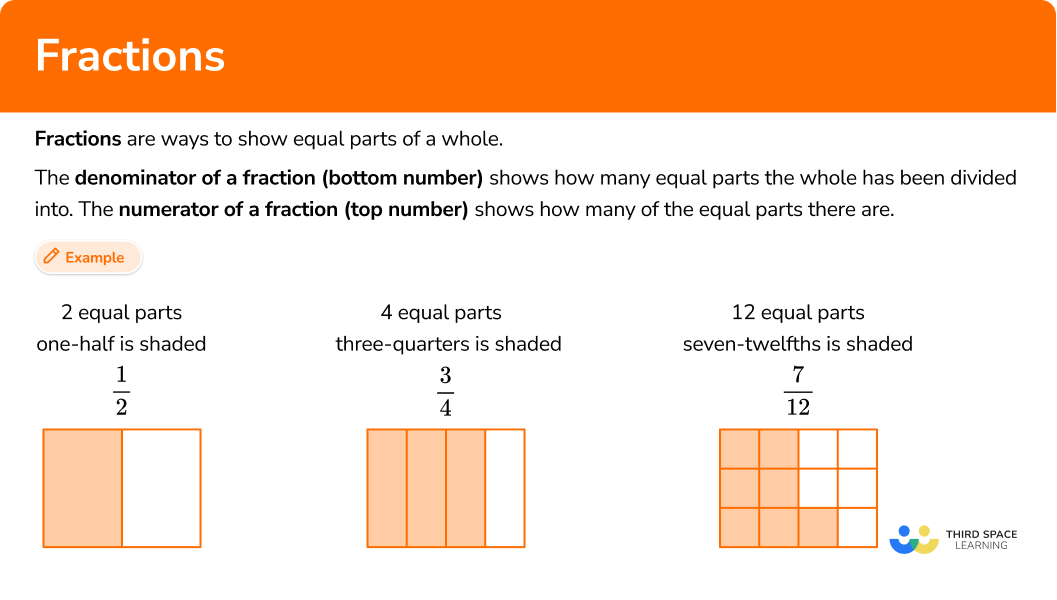## Common Core State Standards

How does this relate to 4th grade math and 5th grade math?

• Grade 4 – Number and Operations – Fractions (4.NF.A.1)
Explain why a fraction \, \cfrac{a}{b} \, is equivalent to a fraction \, \cfrac{(n \, \times \, a)}{(n \, \times \, b)} \, by using visual fraction models, with attention to how the number and size of the parts differ even though the two fractions themselves are the same size. Use this principle to recognize and generate equivalent fractions.

• Grade 4 – Number and Operations – Fractions (4.NF.A.2)
Compare two fractions with different numerators and different denominators, for example, by creating common denominators or numerators, or by comparing to a benchmark fraction such as \, \cfrac{1}{2} \, . Recognize that comparisons are valid only when the two fractions refer to the same whole. Record the results of comparisons with symbols \, > , \, = , \, or \, < , \, and justify the conclusions, for example, by using a visual fraction model.

• Grade 4 – Number and Operations – Fractions (4.NF.B.3)
Understand a fraction \, \cfrac{a}{b} \, with a > 1 as a sum of fractions \, \cfrac{1}{b} \, .

• Grade 4 – Number and Operations – Fractions (4.NF.B.4)
Apply and extend previous understandings of multiplication to multiply a fraction by a whole number.

• Grade 5 – Number and Operations – Fractions (5.NF.A.1)
Add and subtract fractions with unlike denominators (including mixed numbers) by replacing given fractions with equivalent fractions in such a way as to produce an equivalent sum or difference of fractions with like denominators.

• Grade 5 – Number and Operations – Fractions (5.NF.B.3)
Interpret a fraction as division of the numerator by the denominator ( \, \cfrac{a}{b} \, = a \div b). Solve word problems involving division of whole numbers leading to answers in the form of fractions or mixed numbers, for example, by using visual fraction models or equations to represent the problem.

• Grade 5 – Number and Operations – Fractions (5.NF.B.4)
Apply and extend previous understandings of multiplication to multiply a fraction or whole number by a fraction.

• Grade 5 – Number and Operations – Fractions (5.NF.B.7)
Apply and extend previous understandings of division to divide unit fractions by whole numbers and whole numbers by unit fractions.

• Grade 6 – The Number System (6.NS.A.1)
Interpret and compute quotients of fractions, and solve word problems involving division of fractions by fractions, for example, by using visual fraction models and equations to represent the problem.

## How to work with fractions

There are a lot of ways to work with fractions. For more specific step-by-step guides, check out the fraction pages linked in the “What are fractions?” section above or read through the examples below.

## Fractions practice questions

### Example 1: improper fraction to a mixed number with a model

Write the improper fraction \, \cfrac{13}{5} \, as a mixed number.

1. Model the improper fraction.

Draw 3 wholes and divide them equally into fifths. Then shade in 13 parts.

2Count the number of wholes and the fraction left over.

There are 2 wholes (or \, \cfrac{10}{5} \, ) shaded in and there is \, \cfrac{3}{5} \, left over.

3Write the mixed number.

\cfrac{13}{5}=2\cfrac{3}{5}

This can also be solved by dividing the numerator by the denominator:

13 \div 5=2 \, R \, 3 \, or \, 2 \cfrac{3}{5} \, .

### Example 2: compare using common denominators

Compare: \, \cfrac{5}{6} \bigcirc \cfrac{11}{12} \, .

See if the fractions have like denominators.

Make equivalent fractions if needed.

Write the answer using the original fractions.

### Example 3: subtracting fractions with unlike denominators

Solve \, \cfrac{5}{8}-\cfrac{1}{2} \, .

Create common denominators (bottom numbers).

Add or subtract the numerators (top numbers).

### Example 4: proper fractions of numbers

Find \, \cfrac{2}{10} \, of 44.

Convert to a multiplication statement.

Convert the whole number to an improper fraction.

Multiply the numerators together and the denominators together.

### Example 5: multiplying a mixed number by a fraction with the algorithm

Solve \cfrac{3}{12} \times 2 \cfrac{1}{4} \, .

Convert whole numbers and mixed numbers to improper fractions.

Multiply the numerators together.

Multiply the denominators together.

If possible, simplify or convert to a mixed number.

### Example 6: word problem dividing mixed numbers

To make a bracelet, Jenny needs \, \cfrac{2}{5} \, m of string. How many complete bracelets can be made from 3\cfrac{1}{10} \, m of string?

Create an equation to model the problem.

Change any mixed numbers to an improper fraction.

Take the reciprocal of (or flip) the divisor (second fraction).

Change the division sign to a multiplication sign.

Multiply the fractions together.

If possible, simplify or convert to a mixed number.

### Teaching tips for fractions

• Fraction work in 3rd grade centers around understanding through models; particularly area models and number lines. To build on this in 4th grade, always have physical or digital models available for students to use when necessary.

• When using worksheets to teach fraction concepts, make sure that students have ample room to solve since there are many different ways that students can solve. Students may use different types of models (including number lines and area models) or use different equations to solve. They need room to show all of this thinking on paper. Even once students begin to use the algorithm for a fraction concept, they will still need adequate room to solve.

• Fraction worksheets can be useful when students are developing understanding, but once students have a successful strategy and can flexibly operate, incorporate math games or real world projects that allow students to operate with fractions in a variety of situations.

• Highlight patterns within and between different fraction topics as students are learning. Encourage students to be pattern-seekers themselves. This will help them make sense of and understand why the algorithms work.

### Easy mistakes to make

• Not drawing clear models
Models can help students solve fraction problems if they are drawn clearly, but can lead to errors and misconceptions if they are drawn incorrectly. They also become more challenging for students to use as the denominator gets larger. Dividing models into many equal parts leaves room for error. To help avoid this, give students access to physical or digital models that are pre-made.

• Assuming that an improper fraction is smaller than a mixed number
Both improper fractions and mixed numbers include wholes, they are just shown in different ways. Improper fractions have wholes included as part of the numerator, so it is not always clear how many wholes there are. This makes it easier to assume that a mixed number is greater, but that is not always true.
For example,
\cfrac{9}{3} \, is larger than 1 \cfrac{1}{3} \, , because \, \cfrac{9}{3}=3.

• Assuming the fraction with the smallest numbers is the smallest fraction
The relationship between the numerator and denominator is what gives a fraction its value, not just thinking about the numerator and denominator separately.
For example,
\cfrac{3}{5} \, may seem smaller than \, \cfrac{55}{100} \, , since 3 and 5 are smaller numbers, but if you use a common denominator to compare them, you see that \, \cfrac{3}{5} \, is the larger fraction:
\cfrac{3 \, \times \, 20}{5 \, \times \, 20}=\cfrac{60}{100} \,\, and \,\, \cfrac{60}{100} \, > \, \cfrac{55}{100} \, .

• Not using a common denominator when adding or subtracting fractions
When being added or subtracted, fractions must have like denominators.
Equivalent fractions can be used to create a common denominator for adding or subtracting fractions with unlike denominators.
For example,

• Not converting mixed numbers to improper fractions when multiplying or dividing
If you try to multiply or divide mixed numbers it is easy not to do the full calculation. You have to multiply ALL of the first number by ALL of the second number.

If you think about it, the answer should be larger than one of the original numbers and it is not. However, if you convert both mixed numbers to improper fractions and solve, you are multiplying the full values and so you will get the correct answer.

### Practice fractions questions

1. Write the following mixed number as an improper fraction: \, 4\cfrac{11}{12}

\cfrac{15}{12}\cfrac{48}{12}\cfrac{59}{12}\cfrac{59}{4}Multiply the denominator by the whole number.12 \times 4=48

Add the product to the numerator and keep the same denominator.48 + 11 = 59

The new numerator is 59 and the denominator is still 12.

4 \cfrac{11}{12}=\cfrac{59}{12}

2.Order the fractions from greatest to least.All the denominators (2, 3, 5 , and 10) have a multiple of 30, so you can use 30 as the common denominator.

\cfrac{3 \, \times \, 5}{6 \, \times \, 5}=\cfrac{15}{30}

\cfrac{1 \, \times \, 10}{3 \, \times \, 10}=\cfrac{10}{30}

\cfrac{3 \, \times \, 3}{10 \, \times \, 3}=\cfrac{9}{30}

\cfrac{2 \, \times \, 6}{5 \, \times \, 6}=\cfrac{12}{30}

Here are the equivalent fractions with the common denominator of 30:

Now that the denominators are the same, you can order them from least to greatest by the numerator:

Rewrite the fractions in their original form.

3.Solve 3 \cfrac{5}{10}+4 \cfrac{6}{10} \, .

7 \cfrac{11}{20}7 \cfrac{1}{10}8 \cfrac{9}{10}8 \cfrac{1}{10}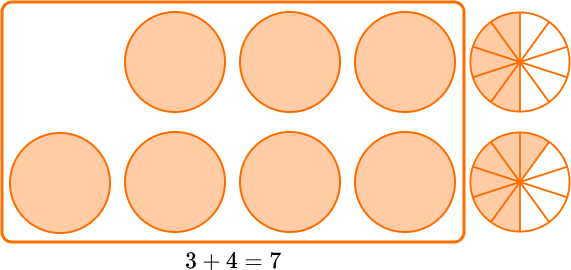Since the denominators are the same, the parts are the same size. You add to see how many parts there are in total: 5 + 6 = 11.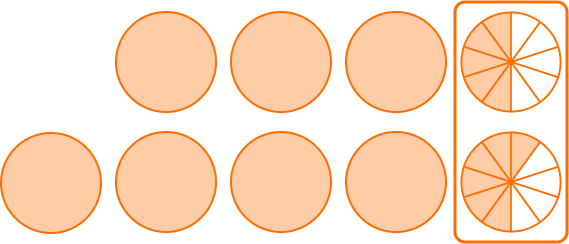There are 11 parts. But what size are the parts? They are still tenths, so the denominator stays the same.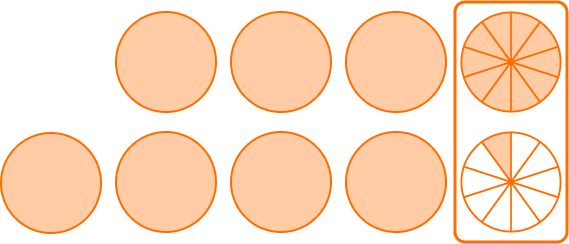\cfrac{5}{10}+\cfrac{6}{10}=\cfrac{11}{10} \, or \, 1 \cfrac{1}{10}

Add the whole numbers and fraction together.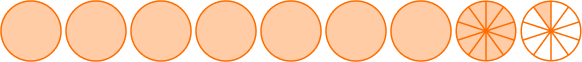7+1 \cfrac{1}{10}=8 \cfrac{1}{10}

4.Find \, \cfrac{1}{3} \, of 18.

61554\cfrac{1}{6}\cfrac{1}{3} \, of 18 is \, \cfrac{1}{3} \times 18.

18 as an improper fraction is \,\cfrac{18}{1} \, .

So, \, \cfrac{1}{3} \times \cfrac{18}{1}=\cfrac{18}{3} \, .

To simplify, use the common factor 3.

\cfrac{18 \, \div \, 3}{3 \, \div \, 3}=\cfrac{6}{1}=6

\cfrac{1}{3} \, of 18 is 6.

5. Solve \, 5 \div \cfrac{1}{4} \, .

\cfrac{5}{4}20\cfrac{1}{9}45 wholes into groups of \, \cfrac{1}{4} \, is 20 groups.

You can also solve this with the algorithm:

Convert the whole number to a fraction: 5 = \cfrac{5}{1}

Take the reciprocal of (or flip) the second fraction: \, \cfrac{1}{4} \rightarrow \cfrac{4}{1}

Change division to multiplication: \cfrac{5}{1} \times \cfrac{4}{1}

Multiply: \cfrac{5}{1} \times \cfrac{4}{1}=\cfrac{20}{1}=20

6. A recipe for cookies calls for \, \cfrac{3}{4} \, cups of sugar. Roy wants to make 3 \cfrac{1}{2} \, recipes of cookies. How many cups of sugar will he need?

4 \cfrac{1}{4} \, cups2 \cfrac{5}{8} \, cups2 \cfrac{3}{4} \, cups4 \cfrac{4}{6} \, cupsSince each recipe has \, \cfrac{3}{4} \, cups of sugar, multiply to solve: \, \cfrac{3}{4} \times 3 \cfrac{1}{2} \, .

Convert the mixed number to an improper fraction.

3 \cfrac{1}{2}=\cfrac{7}{2}Then, multiply the numerators together: \, \cfrac{3}{4} \times \cfrac{7}{2}=\cfrac{21}{} \, .

Then, multiply the denominators together: \, \cfrac{3}{4} \times \cfrac{7}{2}=\cfrac{21}{8} \, .

The numerator is greater than the denominator, so the improper fraction can be converted to a mixed number.

\cfrac{21}{8}=2 \cfrac{5}{8}

Roy needs 2 \cfrac{5}{8} \, cups of sugar.

## Fractions FAQs

Does the answer need to be in lowest terms?

No, these skills do not require students to convert to lowest terms (also known as the simplest form). That said, it is possible that students will be asked to provide an answer in lowest terms. Refer to your state’s standards for specific guidance on when this is appropriate.

Can you compare fractions and mixed numbers to other types of numbers like integers, decimals or percents?

Yes, but just like conversions are required when comparing fractions and mixed numbers, other types of numbers usually also need to be converted for comparisons. For all algorithms, it is important that numbers are in the same form before they are compared.

What is a ‘mixed fraction’ or ‘mixed number form’?

These are both other names for a mixed number and mean the same thing.

How can least common multiple (LCM) and greatest common factor (GCF) be used with fractions?

The least common multiple can be used to find the least common denominator when creating equivalent fractions. The greatest common factor can be used to efficiently simplify fractions to their lowest terms. However, since LCM and GCF are not introduced until 6th grade, younger students should not be expected to use these skills.

## Still stuck?

At Third Space Learning, we specialize in helping teachers and school leaders to provide personalized math support for more of their students through high-quality, online one-on-one math tutoring delivered by subject experts.

Each week, our tutors support thousands of students who are at risk of not meeting their grade-level expectations, and help accelerate their progress and boost their confidence.